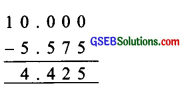# GSEB Solutions Class 6 Maths Chapter 8 Decimals Ex 8.6

Gujarat Board GSEB Textbook Solutions Class 6 Maths Chapter 8 Decimals Ex 8.6 Textbook Questions and Answers.

## Gujarat Board Textbook Solutions Class 6 Maths Chapter 8 Decimals Ex 8.6

Question:
Subtract:
(a) ₹ 18.25 from ₹ 20.75
(b) 202.54 m from 250 m
(c) ₹ 5.36 from ₹ 8.40
(d) 2.051 km from 5.206 km
(e) 0.314 kg from 2.107 kg
Solution:
(a) ₹ 20.75 – ₹ 18.25Here,
2 tens – 1 ten = 1 ten
1 ten = 10 ones
Thus, ₹ 20.75 – ₹ 18.25 = ₹ 2.50

(b) 250 m – 202.54 m
Here, 250 m = 250.00 m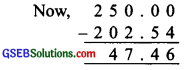Thus, 250 m – 202.54 m = 47.46 m

(c) ₹ 8.40 – ₹ 5.36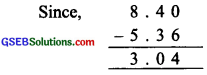∴ ₹ 8.40 – ₹ 5.36 = ₹ 3.04

(d) 5.206 km – 2.051 km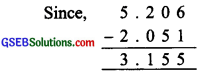Thus, 5.206 km – 2.051 km = 3.155 km

(e) 2.107 kg – 0.314 kg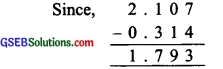Thus, 2.107 kg – 0.314 kg = 1.793 kgQuestion 2.
Find the value of:
(a) 9.756 – 6.28
(b) 21.05 – 15.27
(c) 18.5 – 6.79
(d) 11.6 – 9.847
Solution:
(a) 9.756 – 6.28
Since, 6.28 = 6.280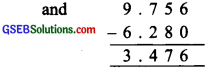Thus, 9.756 – 6.28 = 3.476

(b) 21.05 – 15.27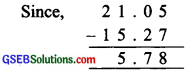∴ 21.05 – 15.27 = 5.78

(c) 18.5 – 6.79
We have 18.5 = 18.50∴ 18.5 – 6.79 = 11.71

(d) 11.6 – 9.847
We have 11.6 = 11.600Thus, 11.6 – 9.847 = 1.753Question 3.
Raju bought a book for ₹ 35.65. He gave ₹ 50 to the shopkeeper. How much money did he get back from the shopkeeper?
Solution:
Money is given to shopkeeper = ₹ 50
Cost of the book = ₹ 35.65
∴ Money got back from the shopkeeper
= ₹ 50 – ₹ 35.65
∵ ₹ 50 = ₹ 50.00
and we have:∴ ₹ 50 – ₹ 35.65 = ₹ 14.35
Thus, money got back = ₹ 14.35Question 4.
Rani had ₹ 18.50. She bought one ice-cream for ₹ 11.75. How much money does she have now?
Solution:
Amount with Rani = ₹ 18.50
Cost of ice-cream = ₹ 11.75
Balance money with Rani = ₹ 18.50 – ₹ 11.75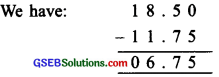Thus, Rani has ₹ 6.75 now.Question 5.
Tina had 20 m 5 cm long cloth. She cuts 4 m 50 cm length of cloth from this for making a curtain. How much cloth is left with her?
Solution:
The total length of cloth with Tina = 20 m 5 cm
Length of the cloth used for curtain = 4 m 50 cm
Length of cloth left with her = 20 m 5 cm – 4 m 50 cm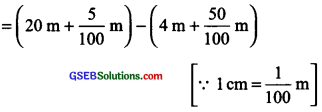= (20 + 0.05) m – (4 + 0.50) m
= 20.05 m – 4.50 m∴ 20.05 m – 4.50 m = 15.55 m
Thus, the length of cloth left = 15.55 mQuestion 6.
Namita travels 20 km 50 m every day. Out of this, she travels 10 km 200 m by bus and the rest by auto. How much distance does she travel by auto?
Solution:
Total distance to be travelled = 20 km 50 m
Distance travelled by bus = 10 km 200 m
The remaining distance to be travelled by auto
= 20 km 50 m – 10 km 200 m
= (20 km + 50 m) – (10 km + 200 m)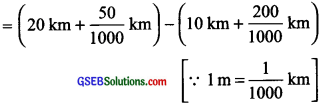= (20 +50 m) – (10 km + 200 m)
= 20.050 km – 10.200 kmThus, the distance travelled by auto = 9.850 kmQuestion 7.
Aakash bought vegetable weighing 10 kg. Out of this, 3 kg 500 g is onions, 2 kg 75 g is tomatoes and the rest is potatoes. What is the weight of the potatoes?
Solution:
Total weight of vegetables = 10 kg
Weight of onions = 3 kg 500 g
Weight of tomatoes = 2 kg 75 g
Total weight of onions + tomatoes = 3 kg 500 g + 2 kg 75 g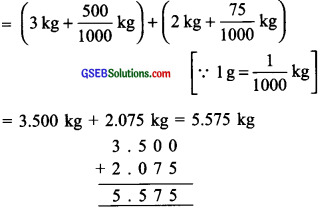Now, weight of potatoes
= 10 kg 0 g – 5.575 kg
= (10,000 – 5.575) kg = 4.425
∴ Weight of potatoes = 4.425 kg